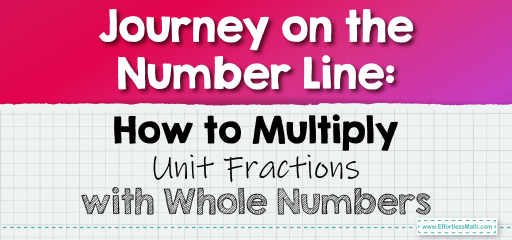# Journey on the Number Line: How to Multiply Unit Fractions with Whole Numbers

Multiplying unit fractions by whole numbers might seem challenging at first, but with the help of a number line, it becomes a visual and intuitive process. A unit fraction is a fraction with a numerator of 1. Let's explore how to use a number line to multiply unit fractions with whole numbers.The Process Explained:

1. Draw a number line.

2. Mark the unit fraction on the number line. 3. Multiply the unit fraction by the whole number by making jumps on the number line.

## Multiplying Unit Fractions by Whole Numbers Using a Number Line

### Example 1:

Multiply $$\frac{1}{4}$$ by 3 using a number line.

Solution Process:

Draw a number line and mark $$\frac{1}{4}$$. Make 3 jumps of $$\frac{1}{4}$$ each.

After 3 jumps, you land on $$\frac{3}{4}$$.

The Absolute Best Book for 5th Grade Students

### Example 2:

Multiply $$\frac{1}{5}$$ by 4 using a number line.

Solution Process:

Draw a number line and mark $$\frac{1}{5}$$. Make 4 jumps of $$\frac{1}{5}$$ each.

After 4 jumps, you land on $$\frac{4}{5}$$.

Using a number line to multiply unit fractions by whole numbers offers a visual representation of the multiplication process. It allows you to see how multiple additions of the same unit fraction lead to the final product. This method is especially helpful for those who are visual learners. So, the next time you’re faced with multiplying a unit fraction by a whole number, grab a number line and visualize your way to the answer!

### Practice Questions:

1. Multiply $$\frac{1}{3}$$ by 2 using a number line.

2. Multiply $$\frac{1}{6}$$ by 5 using a number line.

3. Multiply $$\frac{1}{8}$$ by 3 using a number line.

4. Multiply $$\frac{1}{7}$$ by 4 using a number line.

A Perfect Book for Grade 5 Math Word Problems!

1. $$\frac{2}{3}$$

2. $$\frac{5}{6}$$

3. $$\frac{3}{8}$$

4. $$\frac{4}{7}$$

The Best Math Books for Elementary Students

### What people say about "Journey on the Number Line: How to Multiply Unit Fractions with Whole Numbers - Effortless Math: We Help Students Learn to LOVE Mathematics"?

No one replied yet.

X
30% OFF

Limited time only!

Save Over 30%

SAVE $5 It was$16.99 now it is \$11.99